GeeksforGeeks App
Open AppBrowser
Continue

# Design counter for given sequence

Prerequisite – Counters Problem – Design synchronous counter for sequence: 0 → 1 → 3 → 4 → 5 → 7 → 0, using T flip-flop. Explanation – For given sequence, state transition diagram as following below: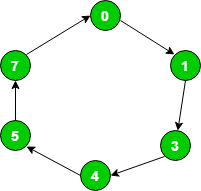State transition table logic:

State transition table for given sequence:

T flip-flop – If value of Q changes either from 0 to 1 or from 1 to 0 then input for T flip-flop is 1 else input value is 0.

Draw input table of all T flip-flops by using the excitation table of T flip-flop. As nature of T flip-flop is toggle in nature. Here, Q3 as Most significant bit and Q1 as least significant bit.

Find value of T3, T2, T1 in terms of Q3, Q2, Q1 using K-Map (Karnaugh Map):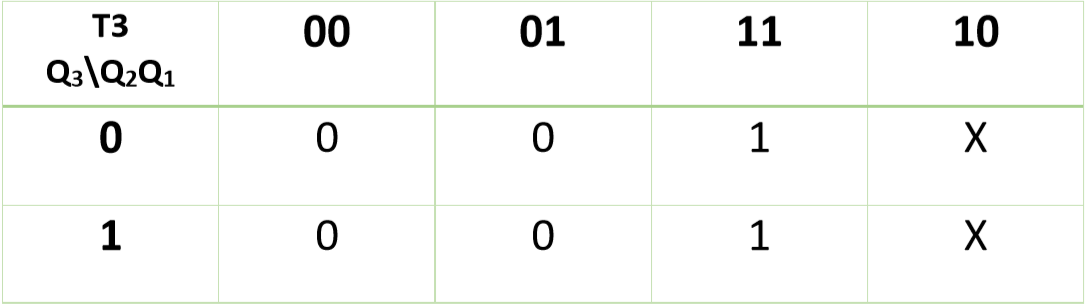Therefore,

`T3 = Q2 `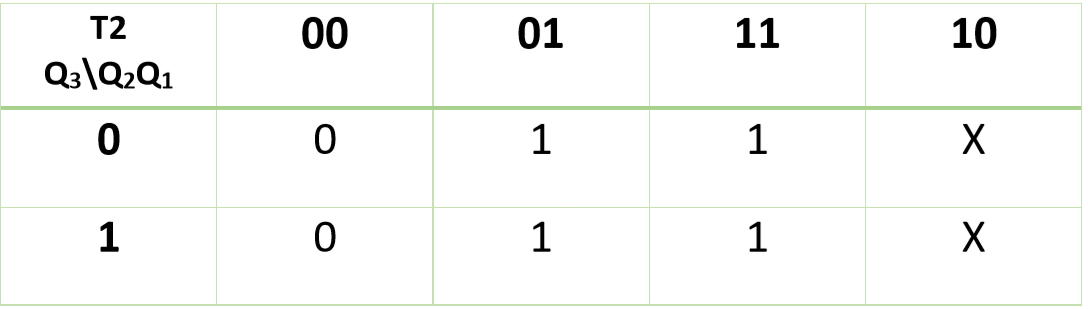Therefore,

`T2 = Q1 `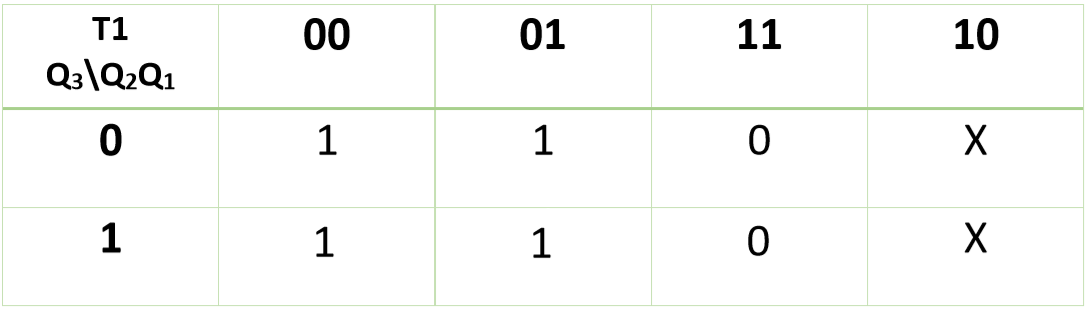Therefore,

`T1 = Q2+Q1'`

Now, you can design required circuit using expressions of K-maps: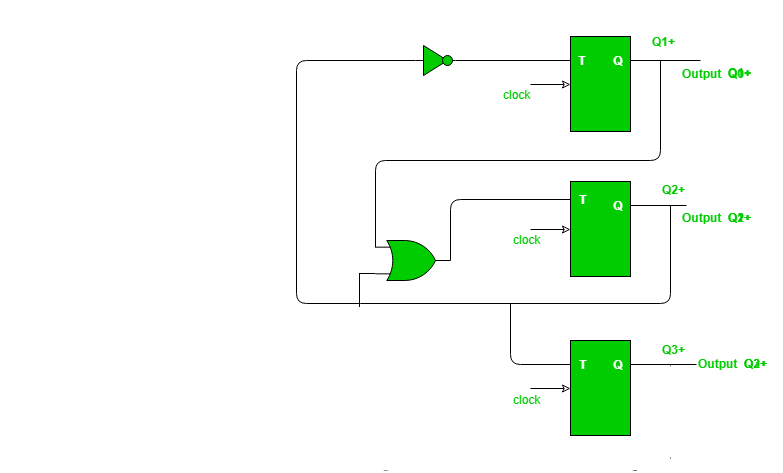My Personal Notes arrow_drop_up